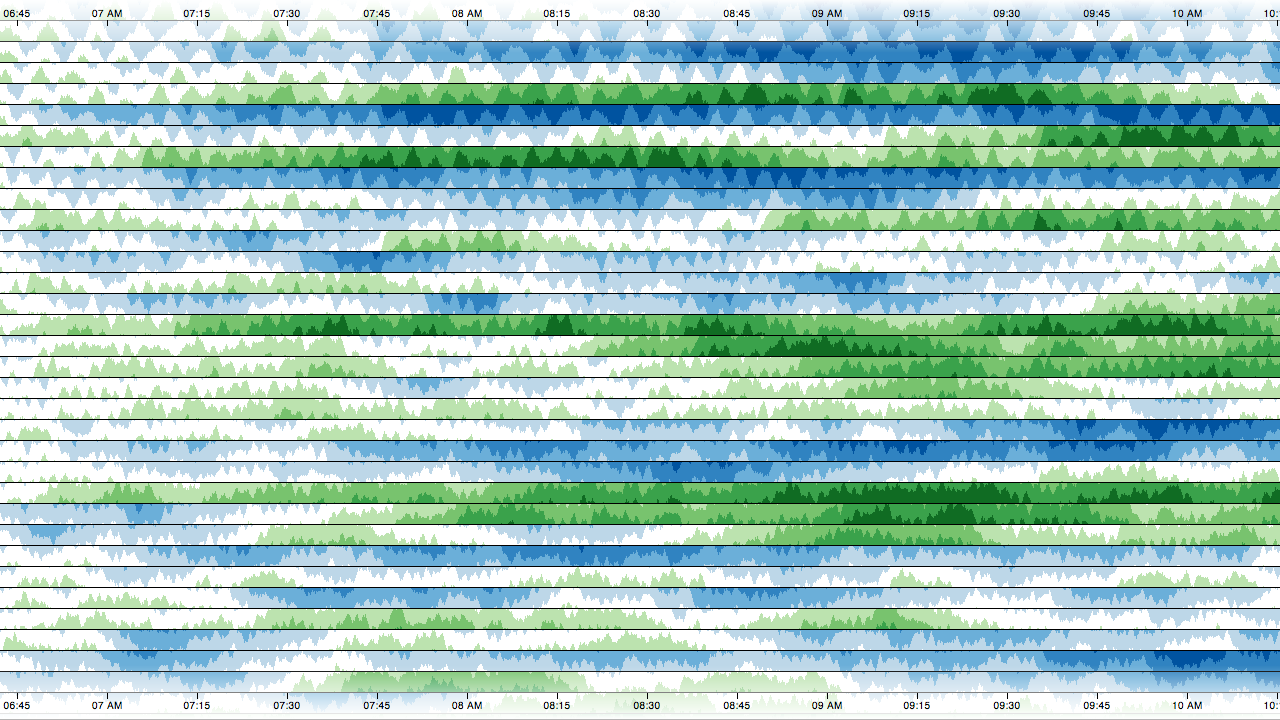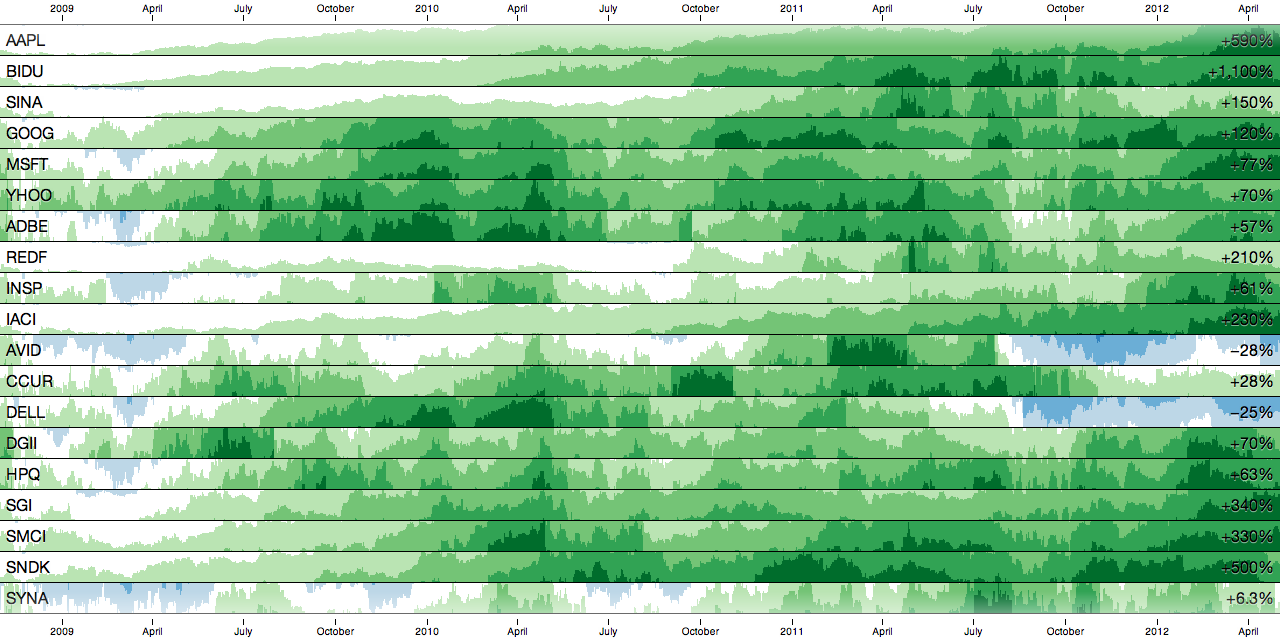# Why do we need visualization*?

## How do we use visualization?

• Anomaly detection - observe abnormal activity
• Capacity planning - extrapolate non-linear trends
• Crisis diagnosis - see interactions between services
• System design - don’t guess; decide empirically

## What are the benefits of visualization?

• Faster diagnosis - reduce impact
• Discovery of unexpected behavior - prevent downtime
• Increased situational awareness - make better decisions

# Why is visualization hard?

## Why is visualization hard?

• Data is not information - transform data to make it meaningful
• Some visual channels are less effective - favor position
• Integrality is hard to avoid - three dimensions max. (XY+Z)
• Not all colors are percieved equally - use color appropriately!
• Doing it right takes time - there’s no free lunch; think it through

## Garish colors can be hard to see.

• Load and render data incrementally.
• Provide details on demand.
• Allow panning & zooming, etc.

# Horizon Charts

1

## Combine position and color to reduce vertical space.# Cubism.js

## A small library, not a system.

``````var context = cubism.context()
.step(1e4) // ten seconds
.size(1440); // four hours``````

## Why a context?

• Avoids distracting redraws - async. fetch, sync. render
• Per-pixel resolution - maximize data density
``````var graphite = context
.graphite("http://example.com");``````

## context.graphite - Define a source for Graphite metrics.

``````var foo = graphite
.metric("sumSeries(foo.*)")
.alias("Foo");``````

## graphite.metric - Fetch data from Graphite.

``````var horizon = context.horizon()
.metric(foo);``````

## Network (5m)

``````var c = a.add(b);      // c = a + b
var c = a.subtract(b); // c = a - b
var c = a.multiply(b); // c = a * b
var c = a.divide(b);   // c = a / b``````

## Metrics are composable, even across sources.

``````var fooLastWeek = foo
.shift(-7 * 24 * 60 * 60 * 1000);

var fooChange = foo
.subtract(fooLastWeek)
.divide(fooLastWeek);``````

## Time-shift metrics to compare against historical data.

``````body.selectAll(".horizon")
.data([foo, bar, baz])
.enter().append("div")
.attr("class", "horizon")
.call(horizon);``````

## Why a library (not a GUI)?

• Small, orthogonal components - assemble via code
• Highly customizable - add interaction, style, embed
• Highly extensible - define new charts & sources
• Built on D3 and web standards - knowledge is power
• Higher power-to-weight ratio - do more with less